# Packing T-joins

 Importance: Medium ✭✭
 Author(s): DeVos, Matt
 Subject: Graph Theory » Coloring » » Edge coloring
 Keywords: packing T-join
 Prize: bottle of wine (DeVos)
 Posted by: mdevos on: March 7th, 2007
Conjecture   There exists a fixed constant(probably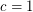suffices) so that every graft with minimum-cut size at leastcontains a-join packing of size at least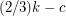.

Definitions: A graft consists of a graphtogether with a distinguished set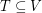of even cardinality. A-cut is an edge-cutofwith the property thatis odd. A-join is a set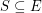with the property that a vertex ofhas odd degree if and only if it is in. A-join packing is a set of pairwise disjoint T-joins.

It is an easy fact that every-join and every-cut intersect in an odd number of elements. It follows easily from this that the maximum size of a-join packing is always less than or equal to the minimum size of a-cut. There is a simple example of a graft with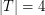with minimum-cut sizewhich contains only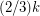disjoint T-joins. The above conjecture asserts that this is essentially the worst case. DeVos and Seymour [DS] have obtained a partial result toward the above conjecture, proving that every graft with minimum-cut sizecontains a-join packing of size at least the floor of.

Definition: We say that a graftis an-graph ifis-regular,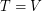, and every-cut of G has size at least.

Conjecture  (Rizzi)   Ifis an-graph, thencontains a-join packing of size at least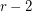.

In an-graph, every perfect matching is a-join, so the above conjecture is true with room to spare for-graphs which are-edge-colorable. Indeed, Seymour had earlier conjectured that every-graph containsdisjoint perfect matchings. This however was disproved by Rizzi [R] who constructed for every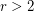an-graph in which every two perfect matchings intersect. Rizzi suggested the above problem as a possible fix for Seymour's conjecture. DeVos and Seymour have proved that every-graph has a-join packing of size at least the floor of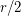.

Definition: Letbe a graph and letbe the set of vertices ofof odd degree. A-join of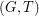is defined to be a postman set.

Note that whenis the set of vertices of odd degree, a cocycle ofis a-cut if and only if it has odd size. Rizzi has shown that the following conjecture is equivalent to the above conjecture in the special case whenis odd.

Conjecture  (The packing postman sets conjecture (Rizzi))   If every odd edge-cut ofhas sizethen the edges ofmay be partitioned intopostman sets.

The Petersen graph (or more generally any non-edge-colorable-graph) shows that the above conjecture would be false with the weaker assumption that every odd edge-cut has size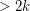. The following conjecture asserts that odd edge-cut sizeis enough (for the same conclusion) if we assume in addition that G has no Petersen minor.

Conjecture  (Conforti, Johnson)   Ifhas no Petersen minor and every odd edge-cut ofhas sizethen the edges ofmay be partitioned intopostman sets.

Gerard Cornuejols [C] has kindly offered \$5000 for a solution to this conjecture. However, it will be tough to find a quick proof since this conjecture does imply the 4-color theorem. Robertson, Seymour, Sanders, and Thomas [RSST] have proved the above conjecture for cubic graphs. Conforti and Johnson [CJ] proved it under the added hypothesis that G has no 4-wheel minor.

## Bibliography

[CJ] M. Conforti and E.L. Johnson, Two min-max theorems for graphs noncontractible to a four wheel, preprint.

[C] G. Cornuejols, Combinatorial Optimization, packing and covering, SIAM, Philadelphia (2001).

[R] R. Rizzi, Indecomposable r-Graphs and Some Other Counterexamples, J. Graph Theory 32 (1999) 1-15. MathSciNet

[RSST] N. Robertson, D.P. Sanders, P.D. Seymour, and R. Thomas, A New Proof of the Four-Color Theorem, Electron. Res. Announc., Am. Math. Soc. 02, no 1 (1996) 17-25.

[S] P.D. Seymour, Some Unsolved Problems on One-Factorizations of Graphs, in Graph Theory and Related Topics, edited by J.A. Bondy and U.S.R. Murty, Academic Press, New York 1979) 367-368.

* indicates original appearance(s) of problem.